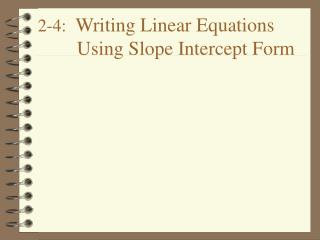DownloadDownload Presentation2-4: Writing Linear Equations Using Slope Intercept Form

# 2-4: Writing Linear Equations Using Slope Intercept Form

Télécharger la présentation## 2-4: Writing Linear Equations Using Slope Intercept Form

- - - - - - - - - - - - - - - - - - - - - - - - - - - E N D - - - - - - - - - - - - - - - - - - - - - - - - - - -
##### Presentation Transcript

1. Slope-Intercept Form • y = mx + b [or f(x) = mx + b] • m is the slope • b is the y-intercept

2. x y Find the Equation of the Line • What is the y-intercept? • b = -2 • What is m? • m = • y = x -2

3. x y Find the Equation of the Line • b = ? • b = 1 • m = ? • m = - • y = -x + 1

4. x y Find the Equation of the Line • b = ? • b = 4 • m = ? • m = 0 • y = 0x+4 • y=4

5. 1) y = -4x + 3 m = -4 b = 3 2) y = 5 - x m = - b = 5 3) 8x + y = m = -8 b = 4) 4x - 2y = 10 m = 2 b = -5 Find the Slope and y-intercept of each Equation:

6. Find the Equation Given the Slope and y-intercept 1) m = -3, b = 1 y = -3x + 1 2) m = -, b = -4 y = - x - 4

7. Given m = -1, (2, 1) First, calculate b by substituting the slope and the coordinates into y = mx + b. y = -1x + b 1 = -1(2) + b 1 = -2 + b 3 = b y = -1x + 3 Find the Equation Given the Slope and a Point

8. Find the Equation GivenTwo Points Given (-1, 3) and (2, 1) First, calculate the slope: Second, find b. Use either point... m = , (2, 1) 1 = (2) + b 1 = + b = b